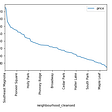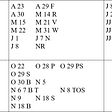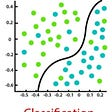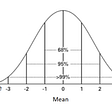# Basic concepts and mathematics

• The input or predictor variable is the variable(s) that help predict the value of the output variable. It is commonly referred to as X.
• The output variable is the variable that we want to predict. It is commonly referred to as Y.
1. It is used to predict the future values of continuous data.
2. Data Should be linear with a single independent and dependent variable.
`###Import necessary librariesimport numpy as npimport pandas as pdfrom matplotlib import pyplot as plt ### Generate 'random' datanp.random.seed(0)### Array of 100 values with mean = 1.5, stddev = 2.5x = 2.5 * np.random.randn(100) + 1.5 # Generate 100 residual terms  res = 0.5 * np.random.randn(100)# Actual values of YY = 2 + 0.3 * X + res                  # Create pandas dataframe to store our X and y valuesdf = pd.DataFrame( {'x': x,     'Y': Y}) # Show the first five rows of our dataframedf.head()`
`# Calculate the mean of X and yxmean = np.mean(x)ymean = np.mean(Y)# Calculate the terms needed for the numerator and denominator of betadf['xycov'] = (df['x'] - xmean) * (df['Y'] - ymean)df['xvar'] = (df['x'] - xmean)**2# Calculate beta and alphab1 = df['xycov'].sum() / df['xvar'].sum()b0 = ymean - (beta * xmean)print(f'b0 = {b0}')print(f'b1 = {b1}')`
`ypred = b0 + b1 x`
`# Plot regression against actual dataplt.figure(figsize=(12, 6))plt.plot(x, ypred)     # regression lineplt.plot(x, Y, 'ro')   # scatter plot showing actual dataplt.title('Actual vs Predicted')plt.xlabel('x')plt.ylabel('Y')plt.show()`
1. Multi Linear Regression is also used to predict the future values of continuous data.
2. Data Should be linear with multiple independent variables and a single dependent variable.
1. Polynomial Regression is used to predict the future values of continuous non-linear data.
2. Data Should be non-linear, with multiple independent variables and a single dependent variable.
1. Multi-variant Regression is used to predict multiple predictors with help of multiple response data.

“Don’t dream to join a tech giant company, dream to make a company gigantic.” — Sai

--

--

--

## More from sai krishna

A Machine Learning technology researcher commencing a new Blog Series to make clear ML concepts Simple and ease for everyone.

Love podcasts or audiobooks? Learn on the go with our new app.

## Building machine learning models to predict Instagram engagement## The Size of The Scuba Diving Industry## Top 10 Facebook Accounts For Data Science…## Insights from Seattle Airbnb data## Time Series Analysis and Forecasting with Python## Isdal Woman: Connecting the Scattered Dots## sai krishna

A Machine Learning technology researcher commencing a new Blog Series to make clear ML concepts Simple and ease for everyone.

## Classification and Regression## Z-score in detail with examples## How to Predict Housing Prices with Linear Regression?